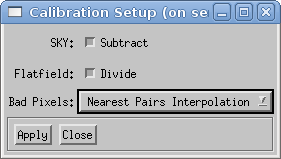# Introduction

The standard calibration algorithm executes the following steps for each raw data image (if requested, see the dialog window in figure 1):

1. Subtracts the sky image from the raw image.
2. Divides the result by the flatfield.
3. The value of each bad pixel is replaced by a value calculated from some neighbor pixels.

Important:

Whether or not you are allowed to subtract the sky background depends on the algorithm used in the reconstruction. For example, in the Richardson-Lucy deconvolution algorithm, the target image must contain the sky background. In this case, the sky background must be kept and subtracted later during the psf preprocessing step.The defect pixel interpolation is provided by an algorithm which uses up to two pairs of good pixels. The pixels of a pair are on the opposite side of a defect pixel, and an interpolation schema calculates the replacement value.